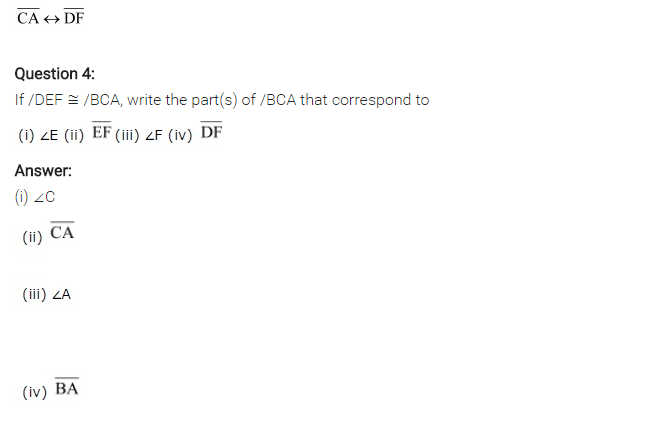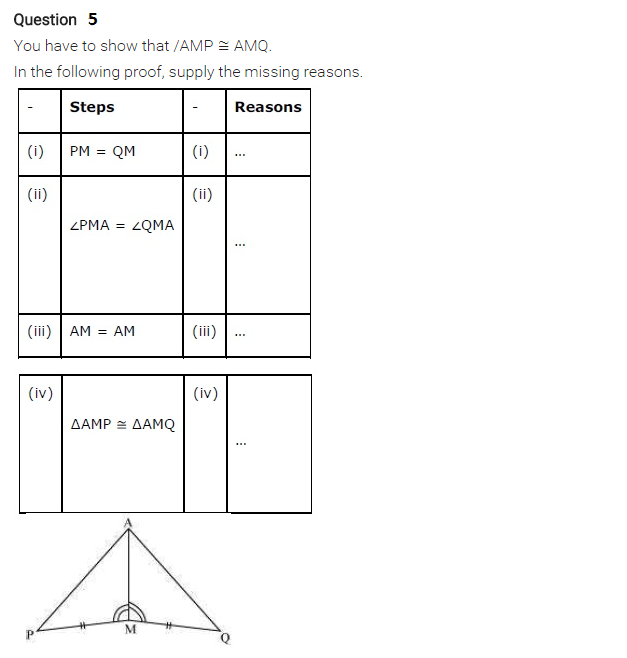# NCERT Solutions for Class 7 Maths Chapter 7 Congruence of Triangles

NCERT Solutions for Class 7 Maths Chapter 7 Congruence of Triangles

### NCERT Solutions for Class 7 Maths Chapter 7 Congruence of Triangles Ex 7.1

NCERT Solutions for Class 7 Maths Chapter 7 Congruence of Triangles Exercise 7.1
Ex 7.1 Class  7 Maths Question 1.
Complete the following statements:
(a) Two line segments are congruent if _______ .
(b) Among the congruent angles, one has a measure of 70°, the measure of the other angle is _______ .
(c) When we write ∠A = ∠B, we actually mean
Solution:
(a) they have the same length
(b) 70°
(c) m∠A = m ∠B

Ex 7.1 Class  7 Maths Question 2.
Give any two real life examples for congruent shapes.
Solution:

• Sharing blades of the same brand.
• Biscuits of the same packets.

Ex 7.1 Class  7 Maths Question 3.
If ∆ABC ≅ ∆FED under the correspondence ABC ⟷ FED, write all the corresponding congruent parts of the triangles.
Solution:
Given: ∆ABC = ∆FED
and ABC ⟷ FEDEx 7.1 Class  7 Maths Question 4.
If ∆DEF ≅ ∆BCA. Write the part of ABCA that correspond toSolution:
Given: ∆DEF ≅ ∆BCA
(i) ∠E ⟷ ∠C
(ii) ∠F ⟷ ∠A
(iii) EF ⟷ ZA
(iv) DF ⟷ BA## SabDekho

The Complete Educational Website